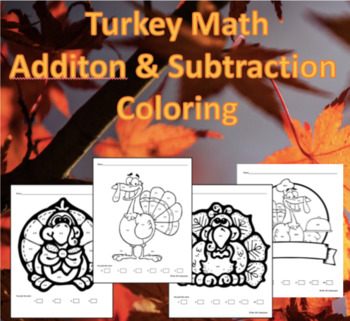# Turkey Math Addition & Subtraction ColoringSubject
Resource Type
Format
PDF (303 KB|4 pages)
Standards
\$2.00
• Product Description
• Standards

These coloring sheets allow students to choose the color and create a key for each sum or difference. Students can choose colors of their choice for any parts of the picture that does not have a math fact. There are a total of 4 sheets that include the following:

Subtraction with a difference of 5 or less

Subtraction with differences between 5-10

Ms. M's Classroom

Check out my other products:

Dollar Products

Literacy Resources

Math Games

Science Resources

Tools for Goal Setting and Common Core

to see state-specific standards (only available in the US).
Fluently add and subtract within 20 using mental strategies. By end of Grade 2, know from memory all sums of two one-digit numbers.
Understand subtraction as an unknown-addend problem. For example, subtract 10 – 8 by finding the number that makes 10 when added to 8.
Apply properties of operations as strategies to add and subtract. If 8 + 3 = 11 is known, then 3 + 8 = 11 is also known. (Commutative property of addition.) To add 2 + 6 + 4, the second two numbers can be added to make a ten, so 2 + 6 + 4 = 2 + 10 = 12. (Associative property of addition.)
Fluently add and subtract within 5.
For any number from 1 to 9, find the number that makes 10 when added to the given number, e.g., by using objects or drawings, and record the answer with a drawing or equation.
Total Pages
4 pages
N/A
Teaching Duration
N/A
Report this Resource to TpT
Reported resources will be reviewed by our team. Report this resource to let us know if this resource violates TpT’s content guidelines.
• Ratings & Reviews
• Q & A

Teachers Pay Teachers is an online marketplace where teachers buy and sell original educational materials.